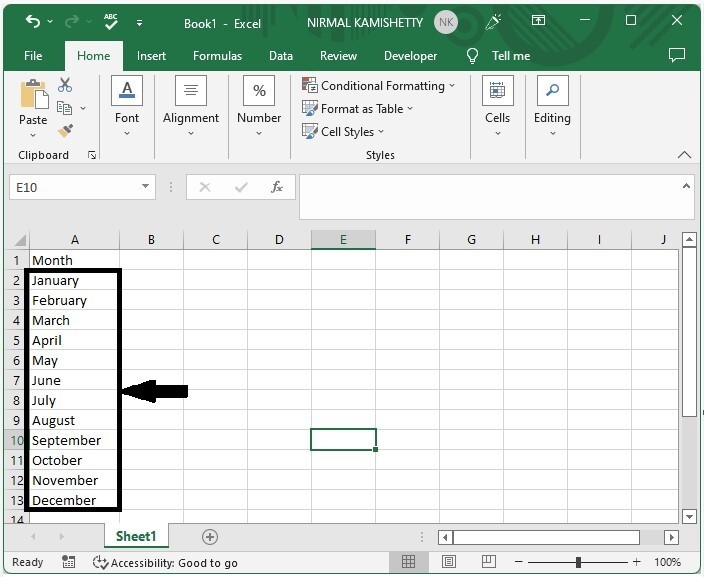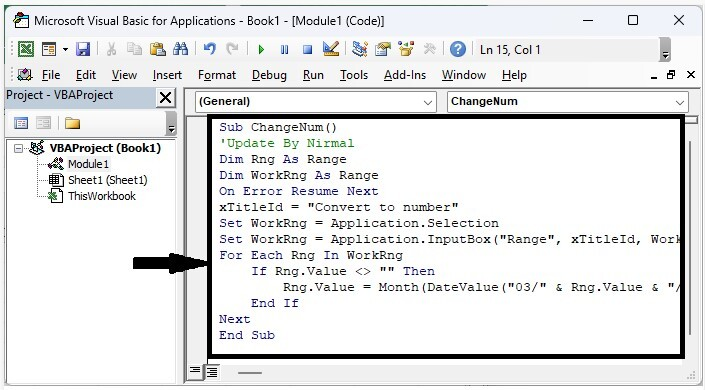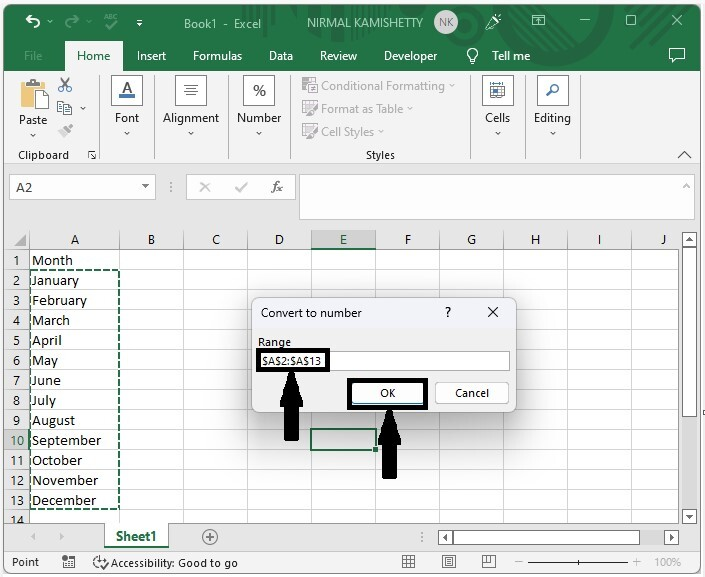# How to Convert Month Name to Number in Excel?

In Excel, you may have needed to convert the month name to a number at times. like January as 1, February as 2, and so on. number is nothing more than their occurrence order number. Read this tutorial to learn how you can convert a month's name to a number and vice versa in Excel. We can complete both tasks using the VBA application. Here we need to use the help of the VBA application, as it can't be completed directly using the formulas.

## Converting Month Name to Number in Excel

Here we will insert a VBA module and then run the code to complete the task. Let us look at a simple procedure for converting month names to numbers in Excel using the vba application.

### Step 1

Consider an excel sheet containing a list of moths, similar to the image below.Now right-click on the sheet name and select view code to open the VBA application, then click on insert and select module.

Right click > view code > insert > module

### Step 2

Then, as shown in the image below, type the following programme into the text box.

### Program

Sub ChangeNum()
'Update By Nirmal
Dim Rng As Range
Dim WorkRng As Range
On Error Resume Next
xTitleId = "Convert to number"
Set WorkRng = Application.Selection
Set WorkRng = Application.InputBox("Range", xTitleId, WorkRng.Address, Type:=8)
For Each Rng In WorkRng
If Rng.Value <> "" Then
Rng.Value = Month(DateValue("03/" & Rng.Value & "/2014"))
End If
Next
End Sub### Step 3

Now save the sheet as a macro-enabled workbook, click F5 to run the code, select the range of values, and click OK.

Save > F5 > Select range > OkIf we need to convert a number to a month name, we can use programme 1.

### Program 1

Sub ChangeMonth()
'Update By Nirmal
Dim Rng As Range
Dim WorkRng As Range
On Error Resume Next
xTitleId = "Change to Month Name"
Set WorkRng = Application.Selection
Set WorkRng = Application.InputBox("Range", xTitleId, WorkRng.Address, Type:=8)
For Each Rng In WorkRng
Rng.Value = VBA.Format(Rng.Value * 29, "mmmm")
Next
End Sub


## Conclusion

We used a simple example in this tutorial to show how to convert month name to number in Excel.

Updated on: 06-Mar-2023

136 Views